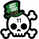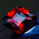58793 vues
Simple indicator to visualize Transient Zones (TZ) and Potential Transient Zones (PTZ).
The last 3 numbers in the header represent: probability of true TZ (black), probability of PTZ (navy) and probability of PTZ resolving (gray).

Version 1.1:
- Added TZ/PTZ occurrence probability thanks to SPYderCrusher

Todo:
- Mid bar transient zones detection
- Draw rectangles for zones (once rectangle drawing gets implemented in pine script) or use dot/circle lines
- Mark forming PTZs more clearly

Previous version 1.0:
```//By Jurij w/ TZ percent occurrence by SPYderCrusher
study("Transient Zones v1.1", "TZ", overlay=true)

//inputs
h_left = input(title="H left", type=integer, defval=10)
h_right = input(title="H right", type=integer, defval=10)
sample_period = input(title="Sample bars for % TZ", type=integer, defval=5000)
show_ptz = input(title="Show PTZ", type=bool, defval=true)
show_channel = input(title="Show channel", type=bool, defval=true)

//barCount = nz(barCount) + 1
//check history and realtime PTZ
h_left_low = lowest(h_left)
h_left_high = highest(h_left)
newlow = low <= h_left_low
newhigh = high >= h_left_high
plotshape(newlow and show_ptz, style=shape.triangledown, location=location.belowbar, color=red)
plotshape(newhigh and show_ptz, style=shape.triangleup, location=location.abovebar, color=green)
channel_high = plot(show_channel ? h_left_low : 0, color=silver)
channel_low = plot (show_channel ? h_left_high : 0, color=silver)

//check true TZ back in history
central_bar_low = low[h_right + 1]
central_bar_high = high[h_right + 1]
full_zone_low = lowest(h_left + h_right + 1)
full_zone_high = highest(h_left + h_right + 1)
central_bar_is_highest = central_bar_high >= full_zone_high
central_bar_is_lowest = central_bar_low <= full_zone_low
plotarrow(central_bar_is_highest ? -1 : 0, offset=-h_right-1)
plotarrow(central_bar_is_lowest ? 1 : 0, offset=-h_right-1)

//TZ probability calc
x = central_bar_is_highest ? 1 : 0
high_bar_tz_count = cum(x)

y = central_bar_is_lowest ? 1 : 0
low_bar_tz_count = cum(y)

total_tz = high_bar_tz_count + low_bar_tz_count
percent_tz_high = (high_bar_tz_count / sample_period) * 100
//plot(percent_tz_high, color = lime, transp=100)
percent_tz_low = (low_bar_tz_count / sample_period) * 100
//plot(low_bar_tz_count, color=red, transp=100)
percent_total_tz = (percent_tz_high + percent_tz_low)
plot(percent_total_tz, color=black, transp=100)

//PTZ probability calc
i = newhigh ? 1 : 0
high_bar_ptz_count = cum(i)

j = newlow ? 1 : 0
low_bar_ptz_count = cum(j)

total_ptz = high_bar_ptz_count + low_bar_ptz_count
percent_ptz_high = (high_bar_ptz_count / sample_period) * 100
//plot(percent_ptz_high, color=green, transp=100)
percent_ptz_low = (low_bar_ptz_count / sample_period) * 100
//plot(percent_ptz_low, color=maroon, transp=100)
percent_total_ptz = (percent_ptz_high + percent_ptz_low)
plot(percent_total_ptz, color=navy,  transp=100)

//PTZ resolving probability calc
percent_ptz_resolved = (1 - (total_tz / total_ptz)) * 100
plot(percent_ptz_resolved, color=gray,  transp=100)
```

## CommentairesIs there a way to add an alert to the Red and Green arrows?
Répondreyrainville
@yrainville, Is there an actual script with Transient that actually works for trading all time frames?
RépondreGarethSA
@GarethSA, what do you mean "works for all timeframes"? The script here can be applied to any timeframe you want, as the transient zones concept is equally applicable to all timeframes. Remember, you cannot just say e.g. "when the big red arrow shows up over the current candle, I'm gonna SELL", because this indicator repaints. With the default settings of hright = 10, you get the arrows 10 candles late. Whatever number you pick for the hright value is how late you'll get the big arrows.

This indicator is NOT meant for that. It is used to study the properties of transient and recurrent zones, which you can read more about here: http://penguintraders.com/2014/12/11/transient-recurrent-zones/ and that site's forums in general.

A final word of advice to anyone who wishes to delve deeper into these concepts. Be prepared to spend a lot of time, as it is a time sink. Is there a way to trade profitably with these concepts? Yes (if you really think outside the box). Are there alternate, faster ways to consistency and profitability? Absolutely. If you think you must study this, my advice is to put this topic at the end of your list of things to look into.

Hope this helps :)
Répondre